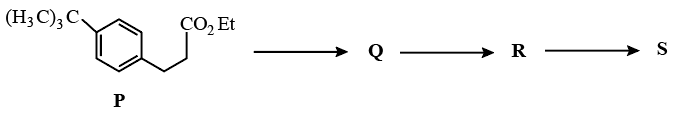### JEE (Advanced) 2017 Paper-2 Question 36

Instructions

The reaction of compound P with $$CH_{3}MgBr$$ (excess) in $$(C_{2}H_{5})_{2}O$$ followed by addition of $$H_{2}O$$ gives Q. The compound Q on treatment with $$H_{2}SO_{4}$$ at $$0^{0}_{C}$$ gives R. The reaction of R with $$CH_{3}COCl$$ in the presence of anhydrous $$AlCl_{3}$$ in $$CH_{2}Cl_{2}$$ followed by treatment with $$H_{2}O$$ produces compound S. [Et in compound P is ethyl group]Question 36